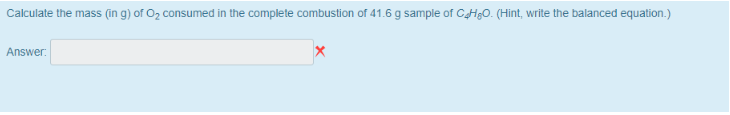# Problem: Calculate the mass (in g) of O2 consumed in the complete combustion of 41.6 g sample of C4H8O. (Hint, write the balanced equation.)

###### FREE Expert Solution
86% (280 ratings)###### Problem Details

Calculate the mass (in g) of O2 consumed in the complete combustion of 41.6 g sample of C4H8O. (Hint, write the balanced equation.)